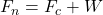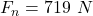## A student of weight 652 N rides a steadily rotating Ferris wheel (the student sits upright). At the highest point, the magnitude of the norm

Question

A student of weight 652 N rides a steadily rotating Ferris wheel (the student sits upright). At the highest point, the magnitude of the normal force on the student from the seat is 585 N. (a) What is the magnitude of at the lowest point

in progress 0
4 months 2021-09-05T10:41:00+00:00 1 Answers 0 views 0

Explanation:

Given

Weight of person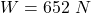At highest point Magnitude of the normal force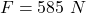net force at highest point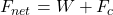where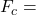centripetal force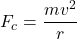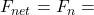Normal Force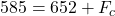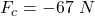Negative sign shows force is in upward direction

At bottom point centripetal force is towards the bottom